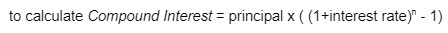Nov. 17, 2022

# How Interest Rates WorksAs you've probably seen the main economic lever used to reduce inflation is to increase interest rates.  So in these inflationary times we see that interest rates are being increased and are going to be increased further until inflation gets back to the magic number of 2%.

But what do increasing interest rates mean for you and your personal finances.  Is it a good thing for you or is it going to cause you pain? Interest rates are something that seem simple but many people fail to understand their impact so it's worth getting up to speed on the basics.

### What is an interest rate?

The amount a lender charges to a borrower which is a percentage of the principal (the amount loaned).

### Who pays interest?

Basically whoever wants the money pays the interest.  Interest rates apply to loans and to money on deposit.  If you take out a loan then you have to pay interest but if you have many on deposit (like a savings account) then you receive interest.  The bottom line here is money isn't free.  If you want money from somebody else you will pay for it, if somebody else wants your money then they will pay for it.

### Types of interest

There are two types of interest: Simple interest and Compound interest. The amount that you pay or receive will depend on how the interest is calculated.

Simple Interest - if you take out a \$10,000 loan from the bank and the interest rate is 4% pa simple interest this means the total interest you will be charged is \$400 at the end of the year.  Therefore your total repayment to the bank is \$10,400.

to calculate Simple Interest = principal x interest rate x time

So let's say you take out a 30 year mortgage of \$300,000 at the same 4% then your interest to pay back is \$300,000 x 4% x 30 = \$360,000.  Now you can see how bank's make their money.

Compound Interest - in this scenario you pay even more interest because as well as paying interest on the principal the borrower also pays interest on the interest! The calculation in this case is based on the number of compounding periods known as n.In the same example of the 30 year mortgage of \$300,000 at 4% using compound interest you will end up paying almost \$700,000 in interest.  That's twice as much as the initial amount borrowed which obviously also needs to be paid back.

### Check the details

Given the huge potential difference between the two types of interest it's important to understand which one applies.  The good news is if you are saving money then Compound Interest is going to work for you and because it has a snowballing effect it can help you build wealth over time.

If you're taking out a loan which incurs Compound Interest then the opposite is true.  Because compound interest increases relative to the number of compounding periods it can be beneficial to try and pay this type of loan off early if the conditions facilitate that.

### APY vs APR?

If you're going to take out a loan you need to know how much interest you're going to pay.  If you put your money in a deposit account you need to know how much you're going to get back.  To make things simple there are two common ways of representing these.

APY is the Annual Percentage Yield. This tells you what interest you will get per year on your savings taking compounding into account.  It's also known as AER or Annual Equivalent Rate.

APR is the Annual Percentage Rate. This tells you what interest you are going to have to pay per year to borrow money from the lender.  APR does not take into effect any compounding of interest.

You can now see how increasing interest rates will impact your savings or borrowings.  The bottom line here is to save as much as you can and borrow as little as you need.

Useful Links: Simple Ways to Save Money, What is Inflation?